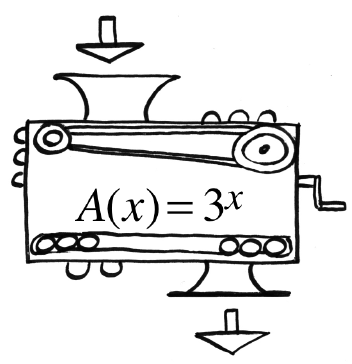### Home > CCA2 > Chapter Ch5 > Lesson 5.1.1 > Problem5-9

5-9.

Antonio’s function machine is shown at right.

1. What is $A(2)$?

$9$

2. If $81$ came out, what was dropped in?

$3^x=81$

Since $9·9=81$ and $3·3=9$, how many threes will you multiply to make $81$?

3. If $8$ came out, what was dropped in? Be accurate to two decimal places.

See part (b).